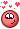## Recommended Posts

Hi every one,

I kinda have an idea to create, though not a good one, but wanted to show it here and ask how would you guys go about this effect in Houdini,

let me know ur thoughts

Edited by angelous4x

##### Share on other sites

Hi @angelous4x  you have an idea I have an idea, + + =  maybe.

transform before /\$PI *2 = x and y

Confuse a cat

play with this and your idea.complex math find

```//q=(1+i+j+k)/2  must be 1 Möbius strip

float cos = v@P.z;
float sin = v@P.x;
float r = v@P.y;

float c_sqr = pow(cos,1) -pow(sin, 1);
float s2 = 1*sin*cos;

vector C = set(c_sqr, 0, s2);
vector B = {0, 1, 0};
vector N = cos * C + sin * B;

v@P = C + r * N;```
```//Sudanese surface.

vector4 gamma(float t) {
return set(cos(t), sin(t), 0, 0);
}

vector4 N(float tau; float t) {
float tau_t = tau * t;
return set(0, 0, cos(tau_t), sin(tau_t));
}

vector4 f_tau(float tau; float x; float y) {
return cos(y) * gamma(x) + sin(y) * N(tau, x);
}

vector sigma(vector4 v) {
return set(v.x / (1 - v.w), v.y / (1 - v.w), v.z / (1 - v.w));
}

float tau = chf("tau");

vector4 q = {1, 1, 1.5, 1};
q /= length(q);

v@P = sigma(qmultiply(f_tau(tau, v@P.x, v@P.y), q));```

or just use file from Matt Ebb a long time ago

Edited by srletak
•2

##### Share on other sites

Here is another approach (definition somewhere from web).

• Using the clifford torus defintion from wiki.
• Using a 4d rotation (in my case between the first and the last component)
• applying a stereographic projection, to map from 4d space back into 3d space

I'm not sure if this is correct, because it looks a bit weird for me ...

clifford_torus.hipnc

##### Share on other sites

I need to work on my math big time. yeah what i was thinking is all in sop level, getting a circle rotating it and getting the closest part of the curve and giving it a value to use a displace by a normal.

## Create an account or sign in to comment

You need to be a member in order to leave a comment

## Create an account

Sign up for a new account in our community. It's easy!

Register a new account